Geometry

# Tools: Using Symmetry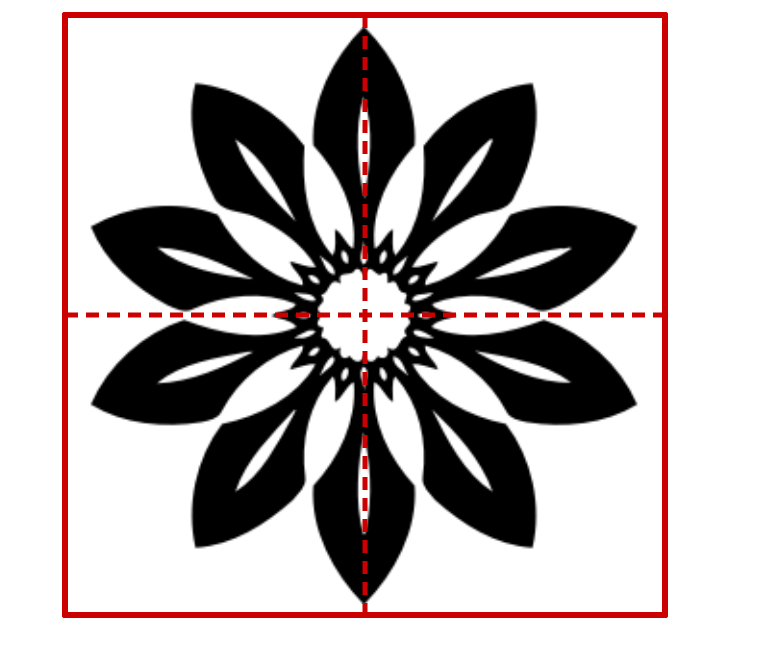Sid wants to calculate the total area of the regions in the red square that are shaded black. He draws lines of symmetry to split the red square into four sections. He plans to calculate the area shaded black within one section, and then multiply that value by four. Will his method work?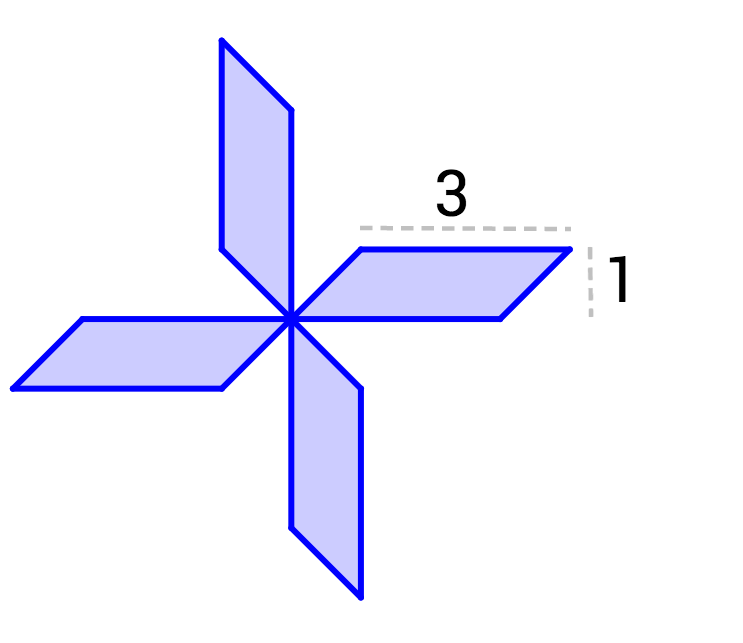If the four parallelograms are congruent, what is the area of the blue figure?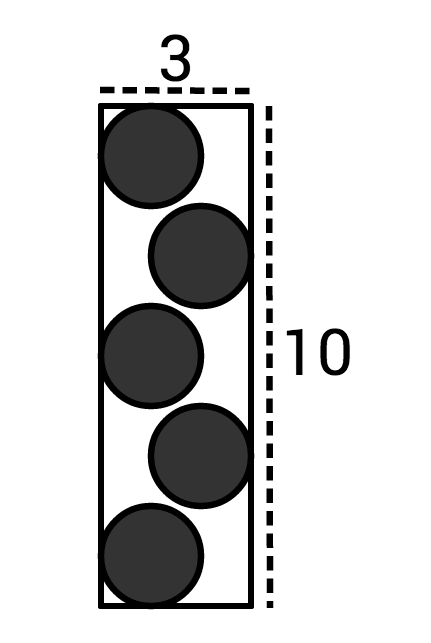Each circle has a radius of 1. Is more of the figure shaded white or black?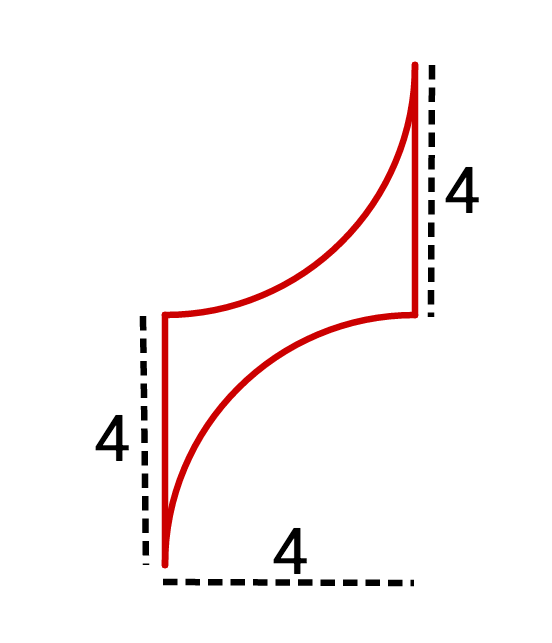What is the area of the red figure? (The arcs are quarter-circles.)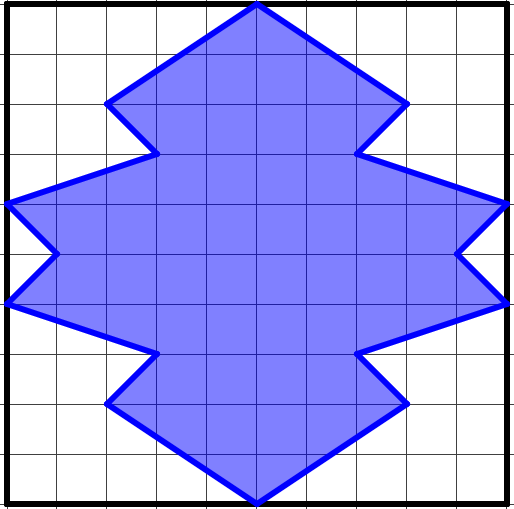Is it possible to draw lines of symmetry on this figure that will split the figure into four congruent pieces?

×